# ML 104: Analyzing Input Data (20 pts extra)

## What You Need

• A Web browser

## Purpose

To practice making simple machine learning code in Python. This project was adapted from chapter 2 of this book:

In a browser, go to

From the menu, click File, "New notebook".

## Checking Package Versions

Execute these commands to check the Python version, and ensure that it is 3.7 or later, and to make sure the scikit-Learn version is 1.0.1 or later. :
```import sys assert sys.version_info >= (3, 7) from packaging import version import sklearn assert version.parse(sklearn.__version__) >= version.parse("1.0.1") ```
As shown below, the commands run without errors, so the versions are OK.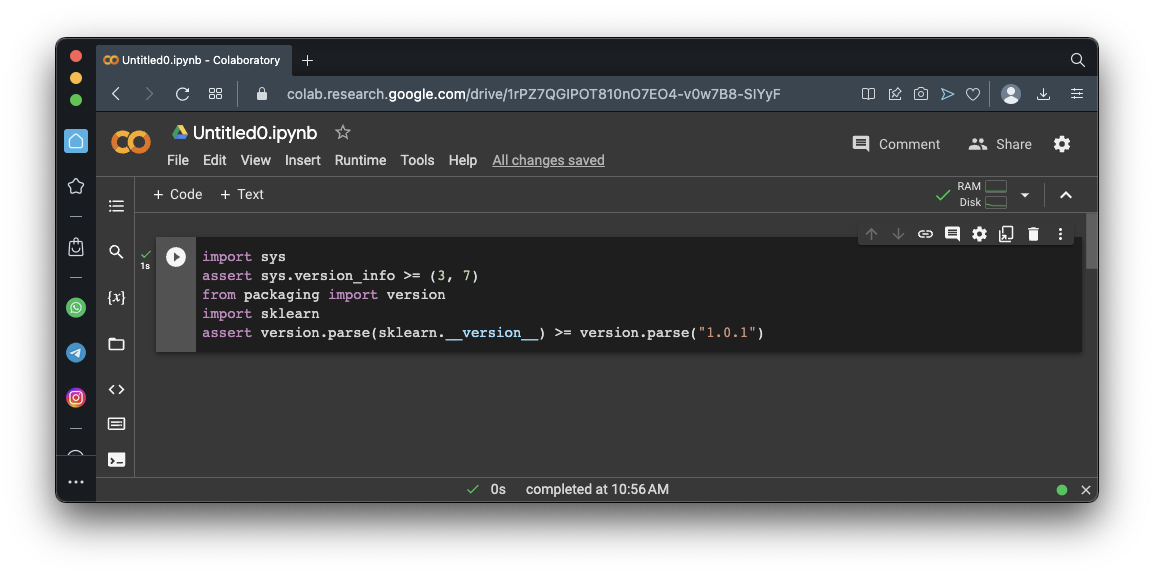# Getting the Data

Execute these commands to download California housing price data, showing housing prices for regions of California we'll call districts.
```from pathlib import Path import pandas as pd import tarfile import urllib.request def load_housing_data(): tarball_path = Path("datasets/housing.tgz") if not tarball_path.is_file(): Path("datasets").mkdir(parents=True, exist_ok=True) url = "https://github.com/ageron/data/raw/main/housing.tgz" urllib.request.urlretrieve(url, tarball_path) with tarfile.open(tarball_path) as housing_tarball: housing_tarball.extractall(path="datasets") return pd.read_csv(Path("datasets/housing/housing.csv")) housing = load_housing_data() housing.info() ```
As shown below, the info() function shows a quick description of the data, showing the number of rows and the names and data types of the columns.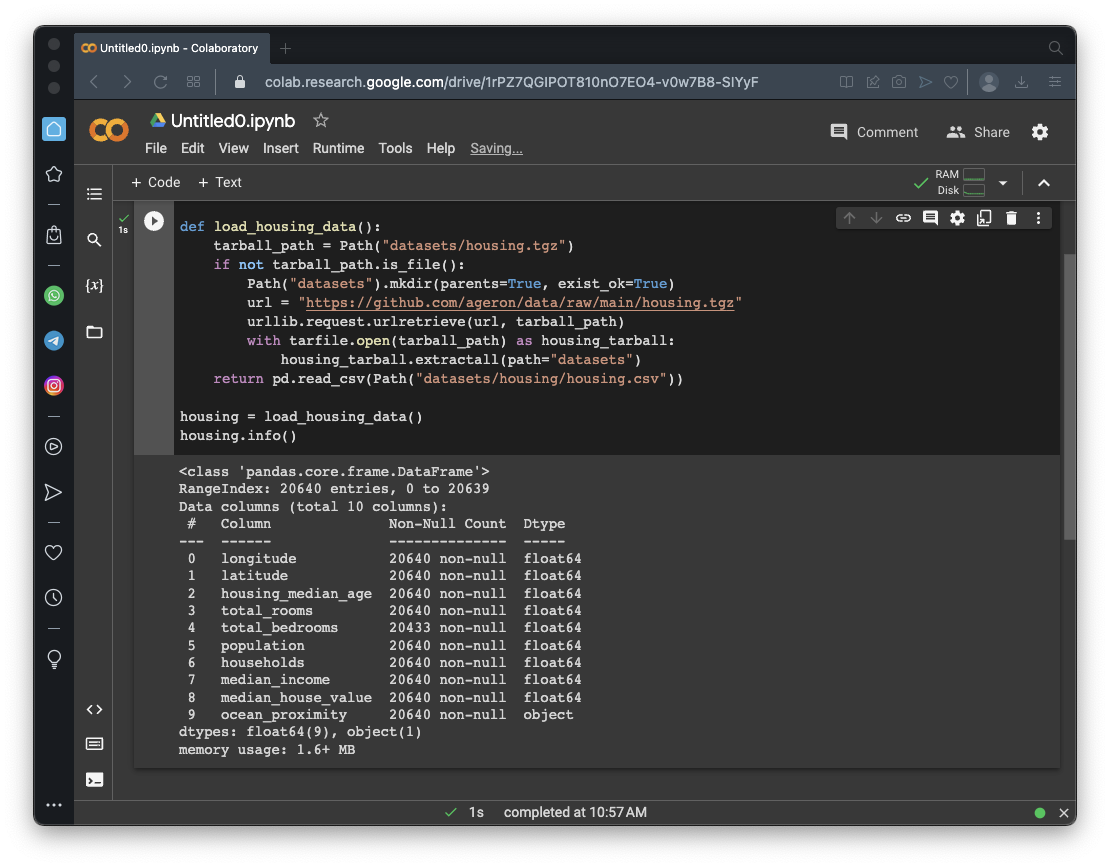Execute this command to see the first 5 rows of data:
```housing.head() ```
As shown below, the values vary, but all the ocean_proximity values are "NEAR BAY".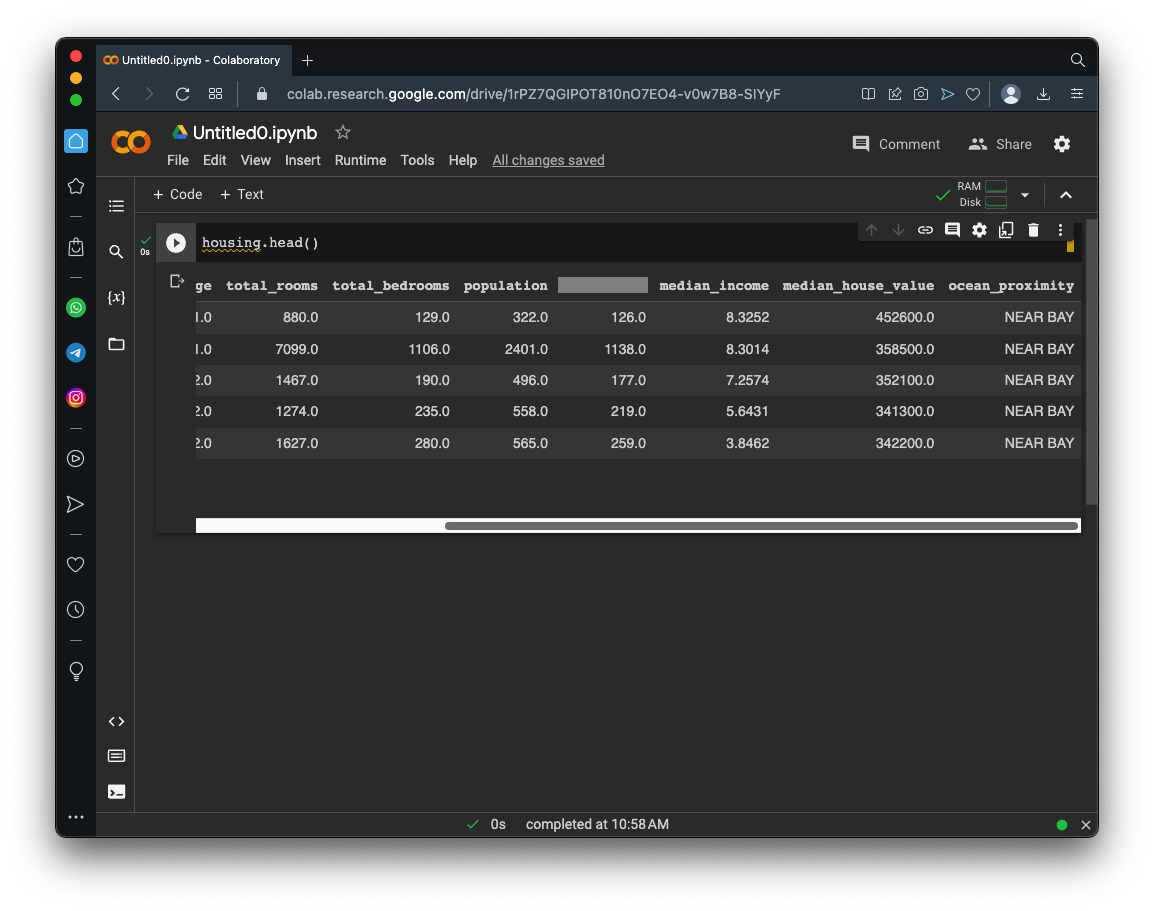## Categories and Counts

Execute this command to see the different values of ocean_proximity and counts of them:
```housing["ocean_proximity"].value_counts() ```
As shown below, there are five possible values.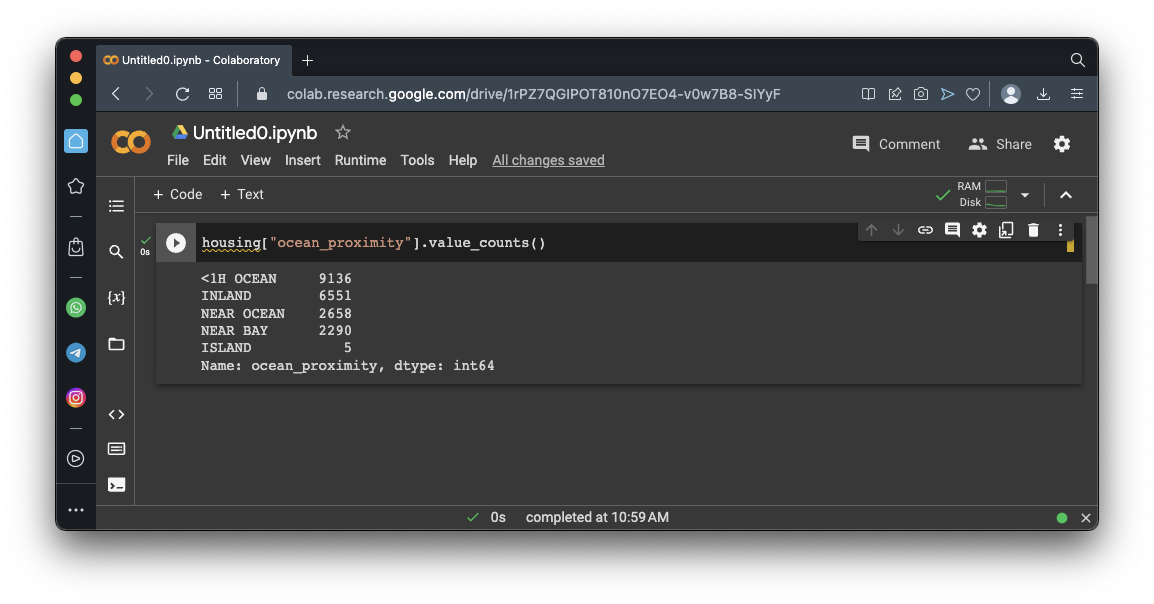## Statistics

Execute this command to see summary statistics of all the numeric fields:
```housing.describe() ```
As shown below, you see the count, mean, and other statistical values for each column.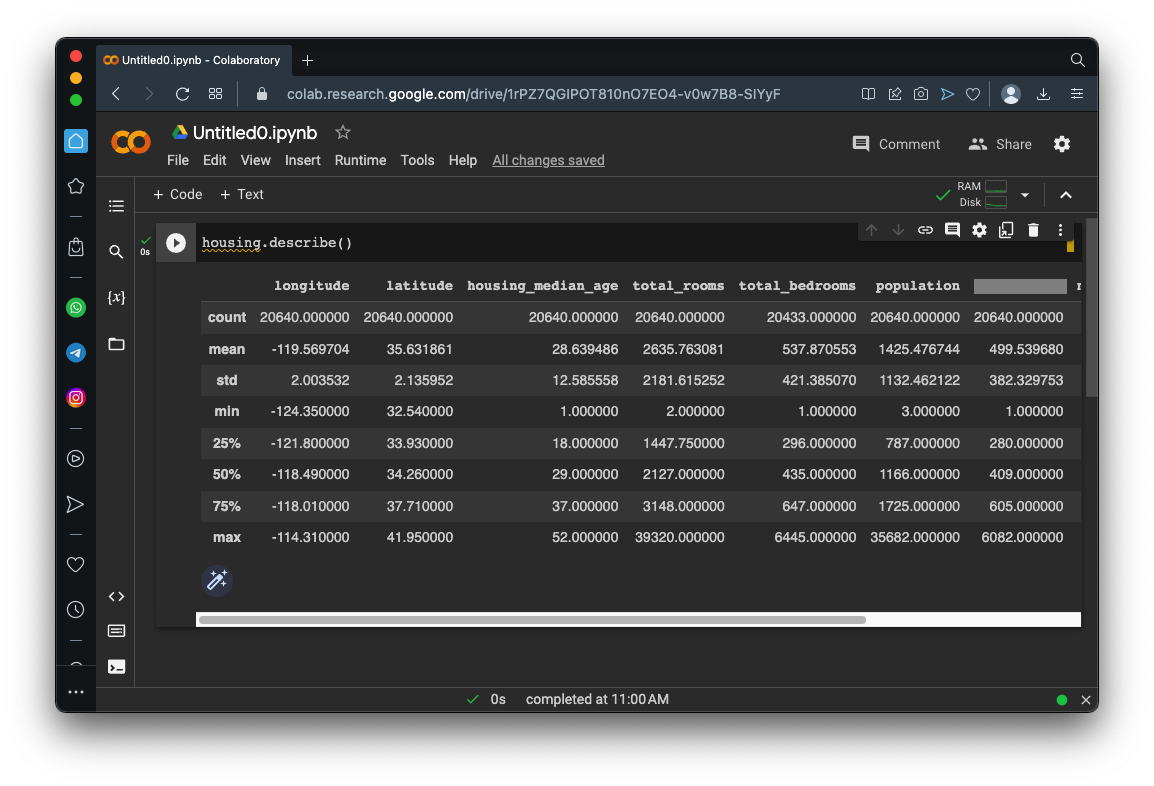## Flag ML 104.1: Histograms (5 pts)

Execute these commands to see histogram plots of each numerical attribute:
```import matplotlib.pyplot as plt housing.hist(bins=50, figsize=(12, 8)) plt.show() ```
As shown below, the plots make it easy to see the distributions of the data.

The flag is covered by a green rectangle in the image below.# Creating Training and Test Sets

## Creating a Test Set Randomly

Execute these commands to pick a random 20% of the data to set aside as a test set:
```import numpy as np def shuffle_and_split_data(data, test_ratio): shuffled_indices = np.random.permutation(len(data)) test_set_size = int(len(data) * test_ratio) test_indices = shuffled_indices[:test_set_size] train_indices = shuffled_indices[test_set_size:] return data.iloc[train_indices], data.iloc[test_indices] train_set, test_set = shuffle_and_split_data(housing, 0.2) print("Training set:", len(train_set)) print("Test set:", len(test_set)) print("Head of test set:") print(test_set.head()) ```
As shown below, the first five rows of the test set appear. Notice the values in the first column--these are the row numbers from the original data set.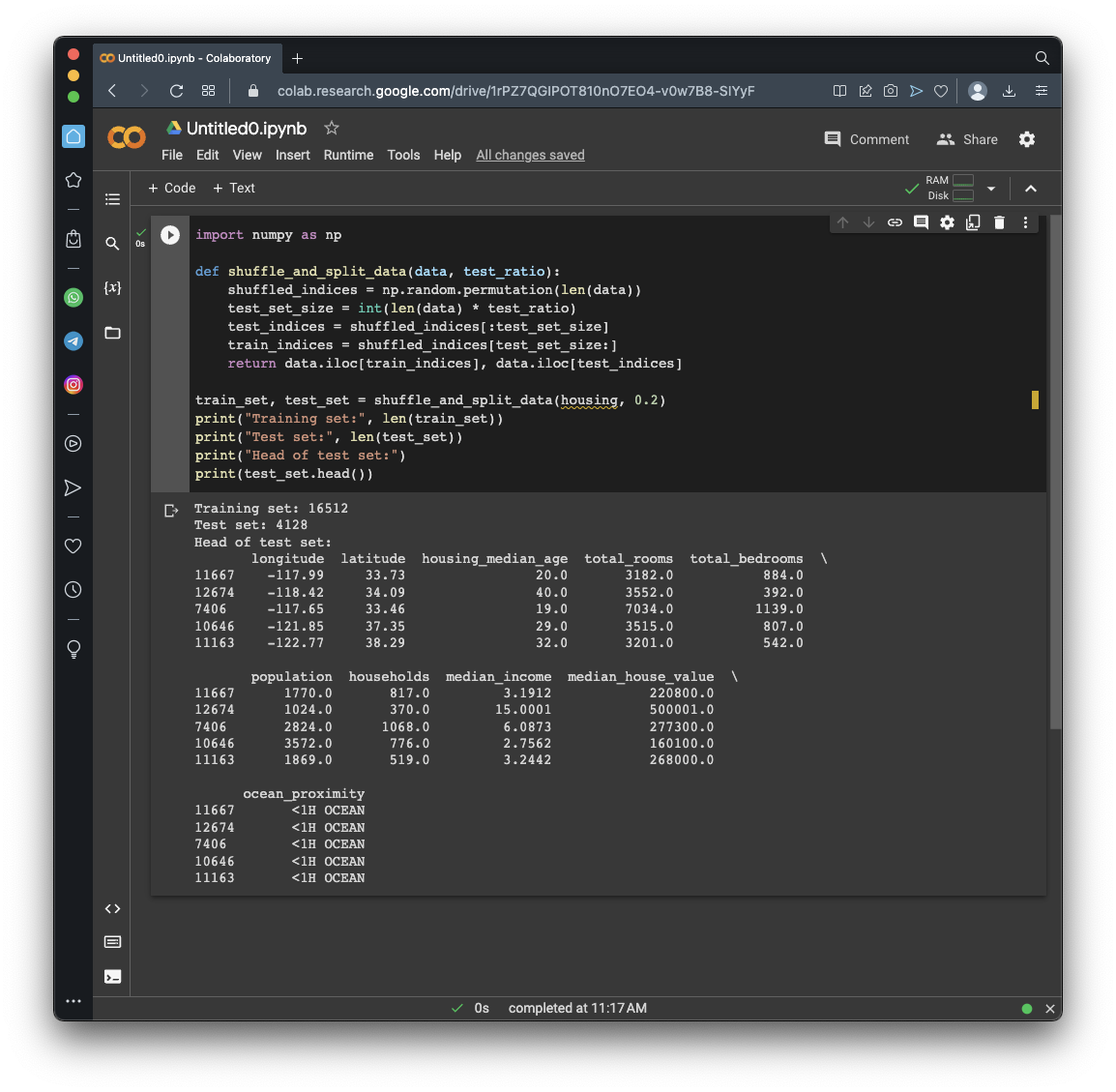Run the previous commands again.

As shown below, different rows are selected each time. This is not the best way to select a training set, because if you run the model many times, the training will see all the data, which is what you want to avoid.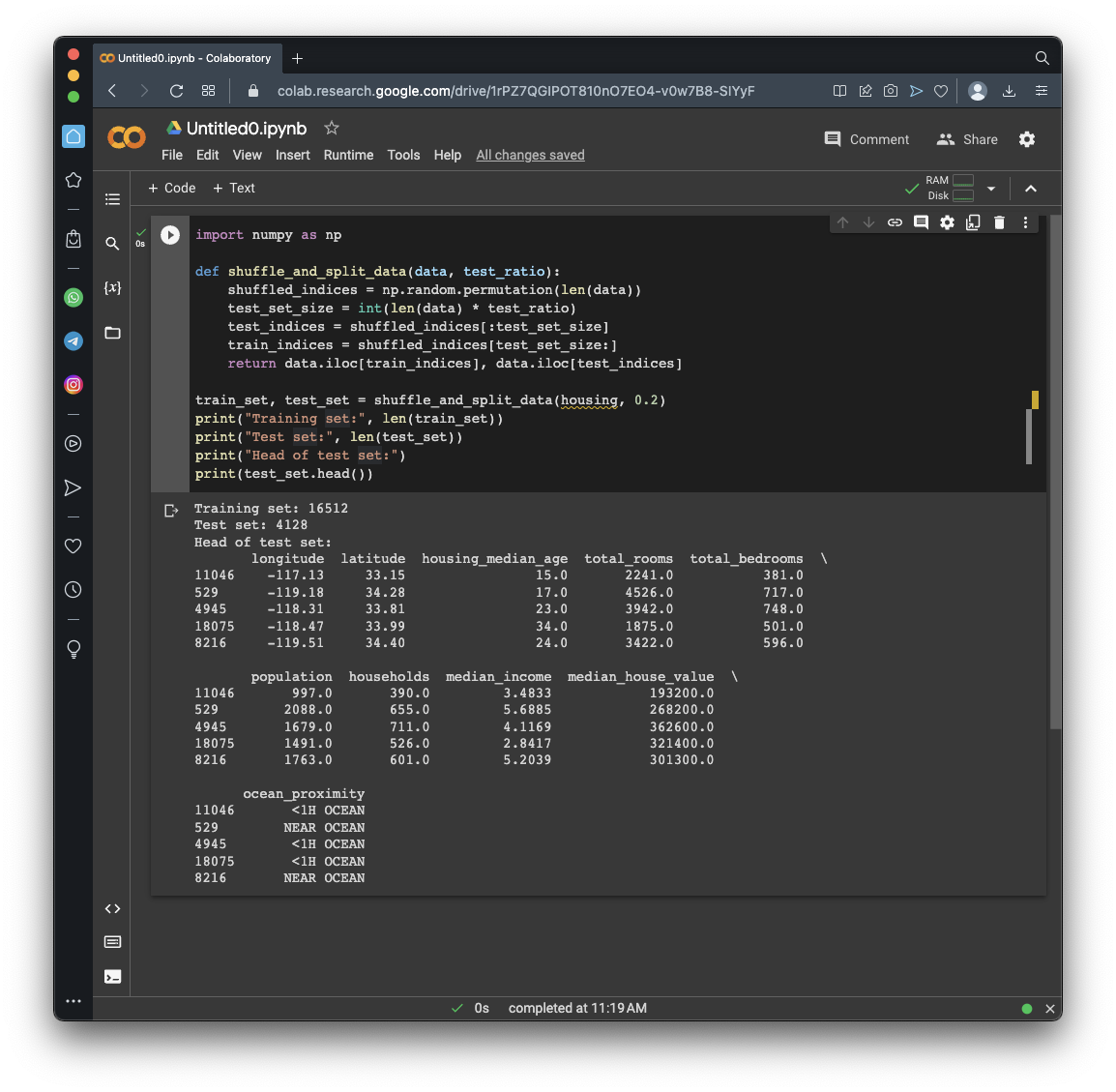## Understanding Biased Sampling

Suppose we are performing a survey of 1000 people. Let's assume that in the U.S., 51.1% of the population are female, and the rest are male. (There are other options in reality, but let's ignore that for now.)

Execute these commands to create 100 random samples of 1000 people and gather the percentage of females in each sample:

```from random import random females = [] for group in range(100): count_females = 0 for i in range(1000): if random() <= 0.511: count_females += 1 females.append(count_females) import matplotlib.pyplot as plt plt.hist(females, 10) plt.show() ```
As shown below, there is a considerable chance that the number of females is 480 or 540, different from the average of 511. A sample like that is biased and will not accurately represent the population.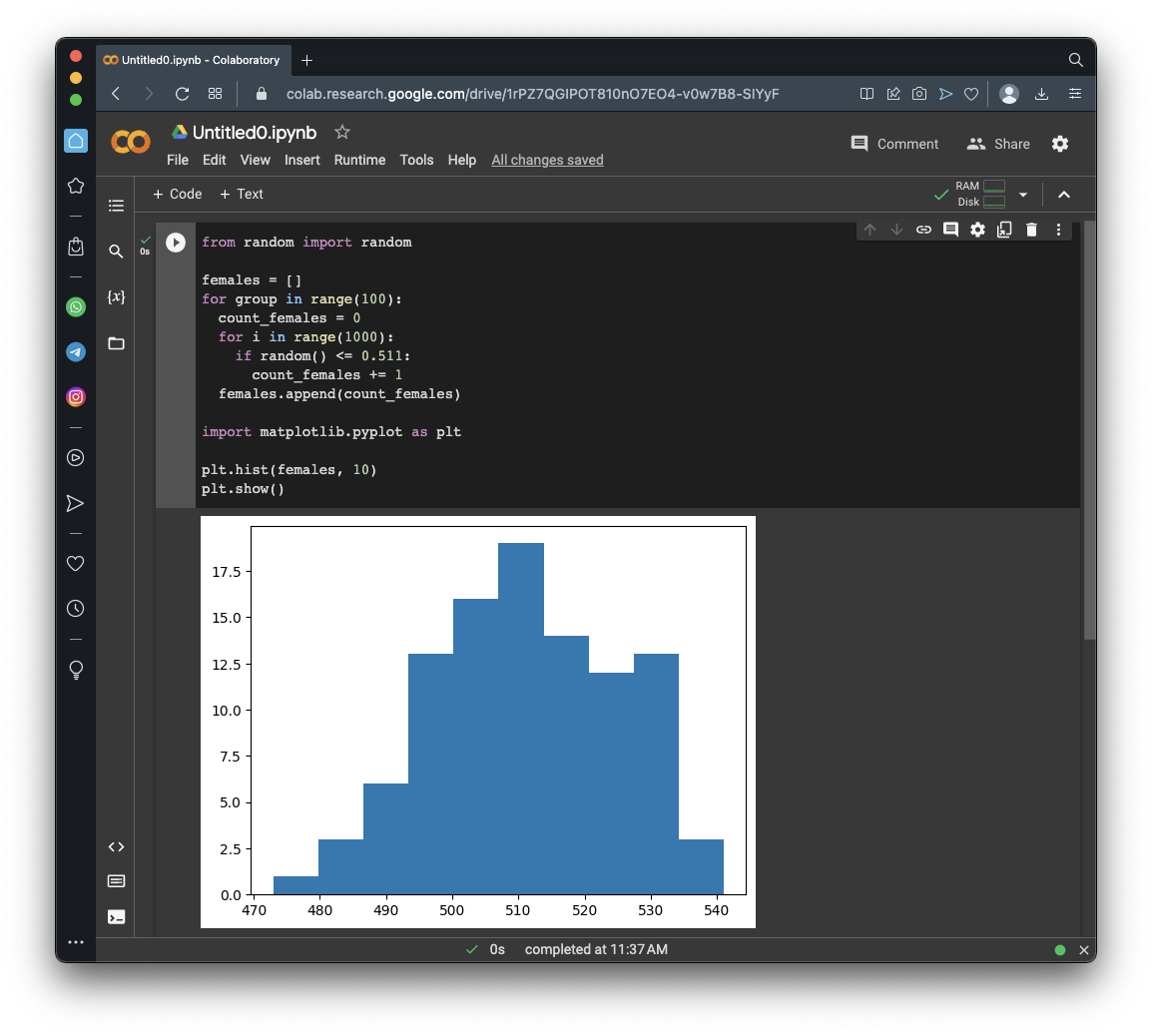## Stratified Sampling

To avoid bias, the population is divided into subgroups called strata and the right number are sampled from each stratum to ensure that the test set represents the population.

In this case, we are training a model to predict a house price in California. We'll postulate that median income is very important to predict house prices, so we want to correctly represent the categories of median income.

As you saw above, in the "Flag ML 104.1: Histograms" section, median income is mostly between 1.5 and 6, but there are some values up to 15.

We'll use these five categories:

1. 0 - 1.5
2. 1.5 - 3
3. 3 - 4.5
4. 4.5 - 6
5. > 6
Execute these commands to define appropriate strata and chart them:
```housing["income_cat"] = pd.cut(housing["median_income"], bins=[0., 1.5, 3.0, 4.5, 6., np.inf], labels=[1, 2, 3, 4, 5]) housing["income_cat"].value_counts().sort_index().plot.bar(rot=0, grid=True) plt.xlabel("Income category") plt.ylabel("Number of districts") plt.show() ```
As shown below, there are reasonable numbers of districts in each category.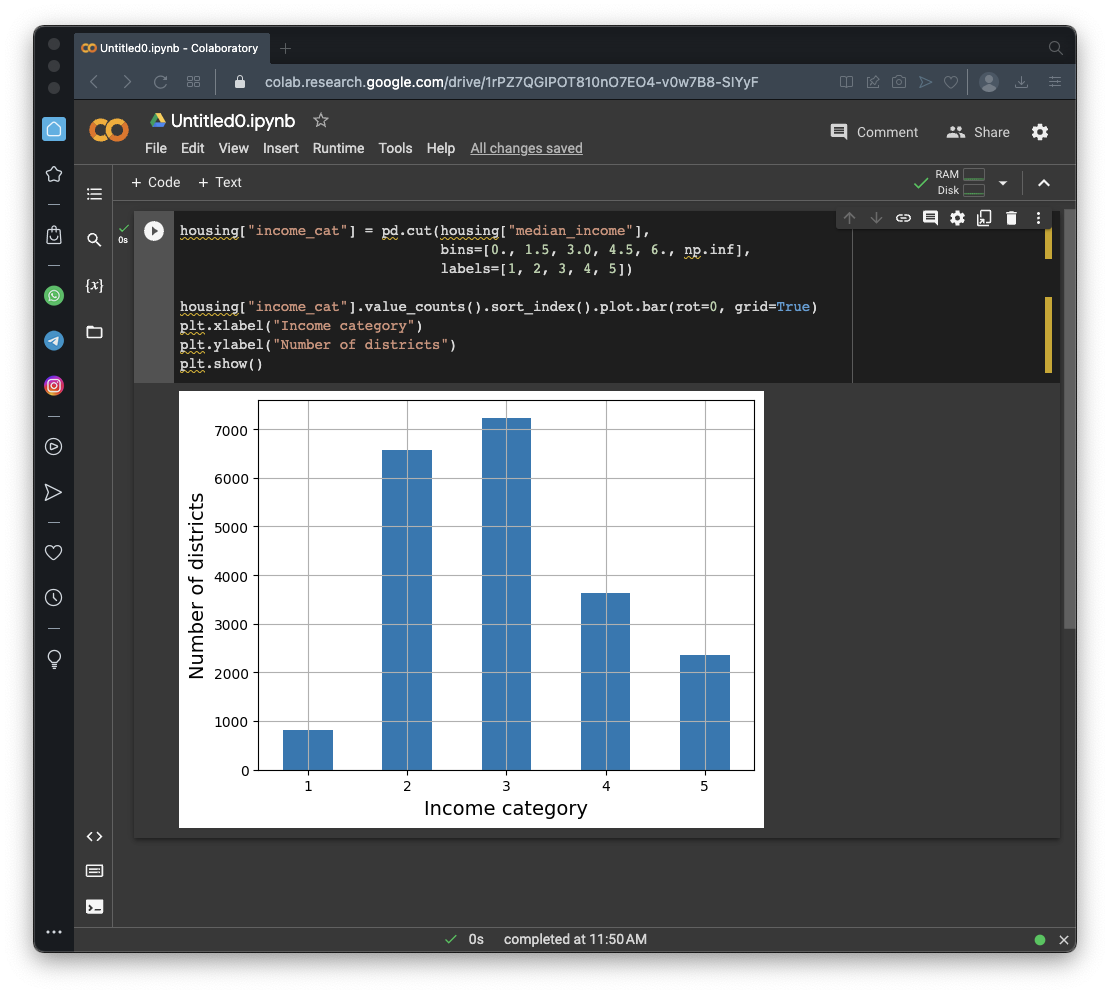## Viewing Records

Execute this command to see the first five rows of the dataset:
```housing.head() ```
As shown below, there's now a column named "income_cat":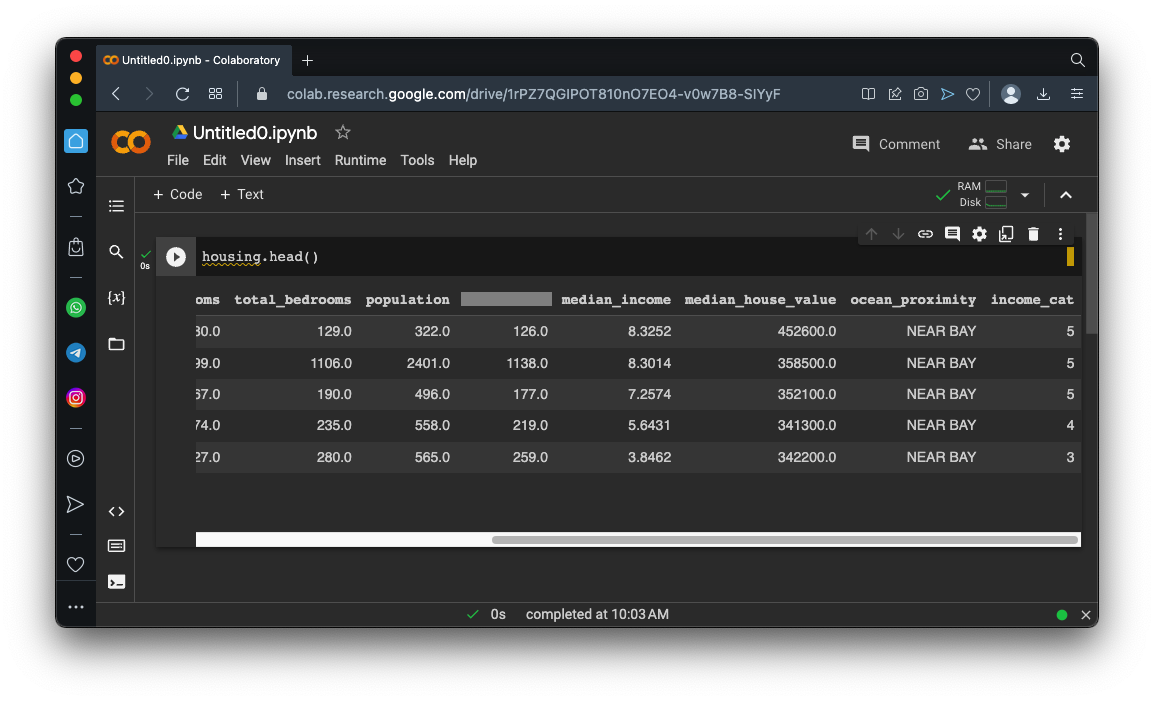## Splitting the Data

Execute these commands to :
```from sklearn.model_selection import train_test_split strat_train_set, strat_test_set = train_test_split( housing, test_size=0.2, stratify=housing["income_cat"], random_state=42) print("Training set:") print(strat_train_set["income_cat"].value_counts() / len(strat_train_set)) print() print("Test set:") print(strat_test_set["income_cat"].value_counts() / len(strat_test_set)) ```
As shown below, the proportion of rows in each income category is very similar in the training set and the testing set. The difference is less than .001 for all categories.## Examining the Unstratified Data Sets

Execute these commands to :
```print("Training set:") print(train_set["income_cat"].value_counts() / len(train_set)) print() print("Test set:") print(test_set["income_cat"].value_counts() / len(test_set)) ```
As shown below, the difference is much larger, up to 0.01. The stratfied sample is a much better representation of the population.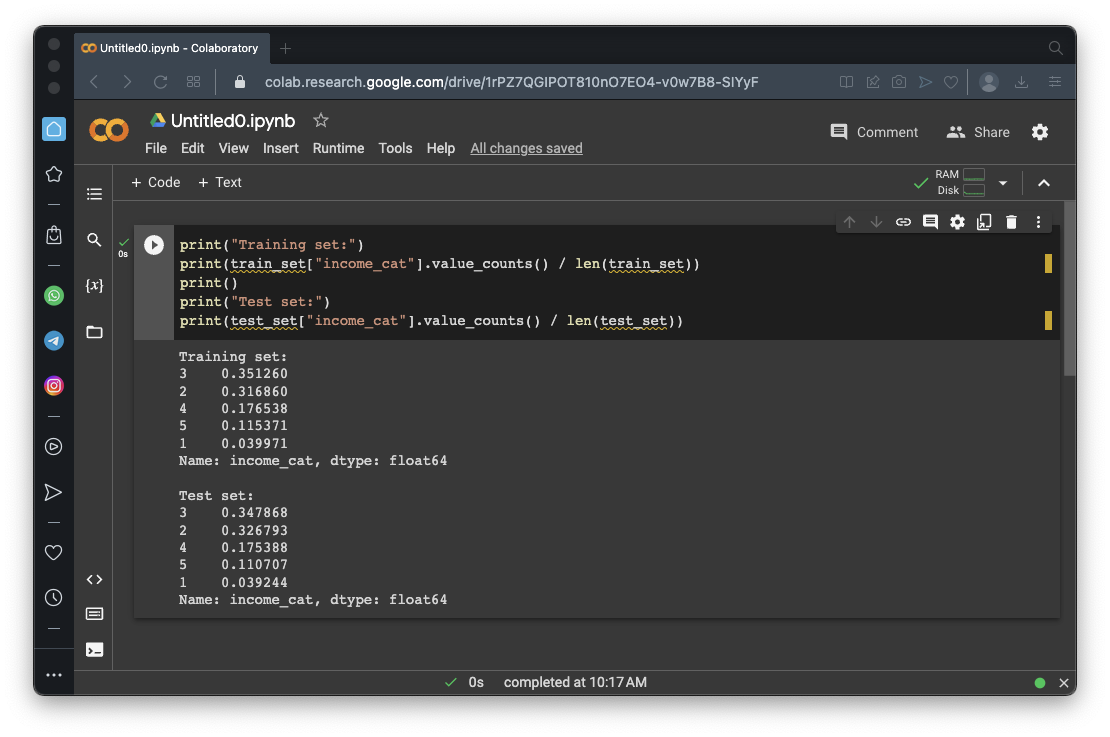## Flag ML 104.2 Removing the "income_cat" column (10 pts)

Execute these commands to remove the income_cat column and see the first five rows to verify that it's gone:
```for set_ in (strat_train_set, strat_test_set): set_.drop("income_cat", axis=1, inplace=True) strat_train_set.head() ```
As shown below, the income_cat column is gone.

The flag is covered by a green rectangle in the image below.# Visualizing the Data

## Plotting by Location

Execute these commands to create a copy of the training set named "housing" and plot the districts by latitude and longitude:
```housing = strat_train_set.copy() housing.plot(kind="scatter", x="longitude", y="latitude", grid=True) plt.show() ```
As shown below, the dots roughly trace out the shape of California, but overlapping dots blot out the details in many regions:## Using Transparent Dots

Execute this command to plot the data using an "alpha" of 0.2, which makes the dots transparent:
```housing.plot(kind="scatter", x="longitude", y="latitude", grid=True, alpha=0.2) plt.show() ```
As shown below, the details are more distinguishable: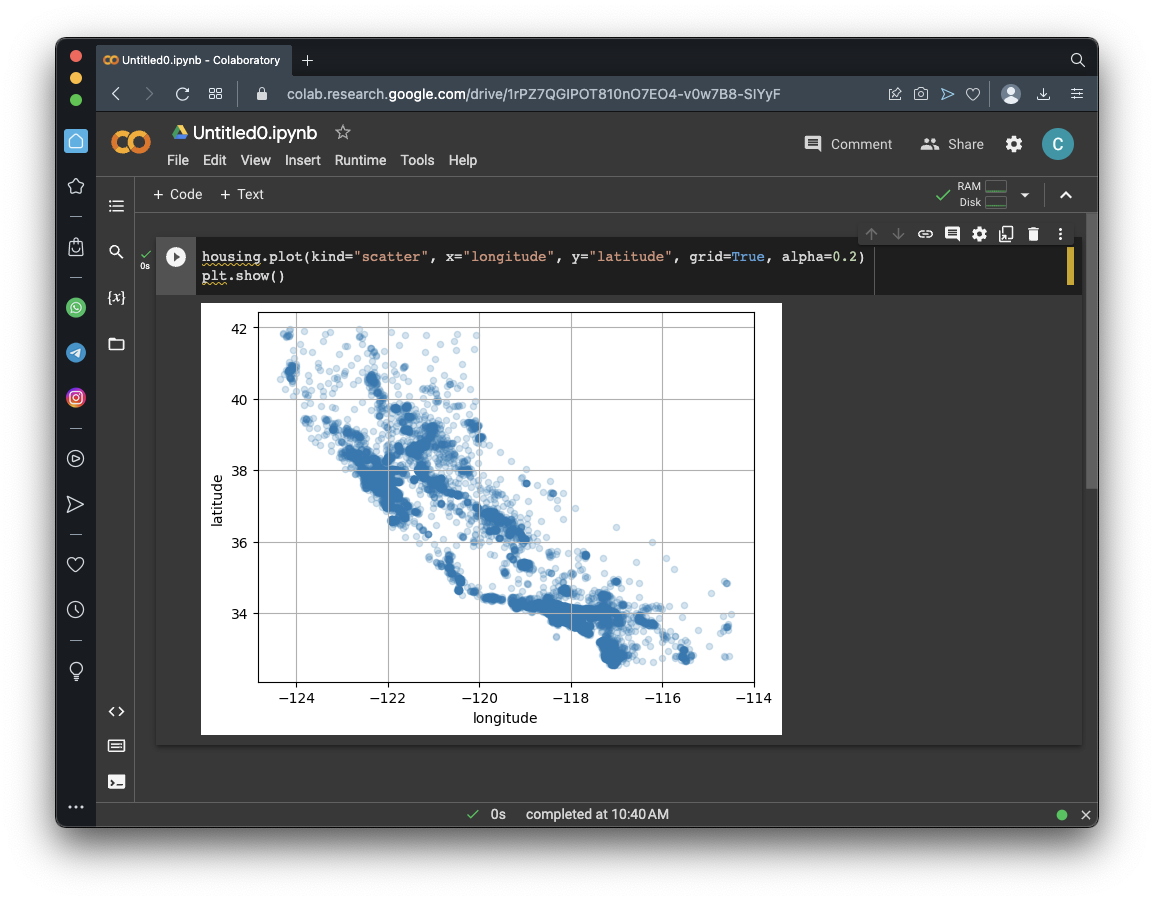## Including Population and Price

Execute these commands to graph using dot size (s) to represent population and color (c) to represent the median_house_value:
```housing.plot(kind="scatter", x="longitude", y="latitude", grid=True, s=housing["population"] / 100, label="population", c="median_house_value", cmap="jet", colorbar=True, legend=True, sharex=False, figsize=(10, 7)) plt.show() ```
As shown below, the most expensive homes are on the coast, in the North near San Francisco, or in the South near Los Angeles.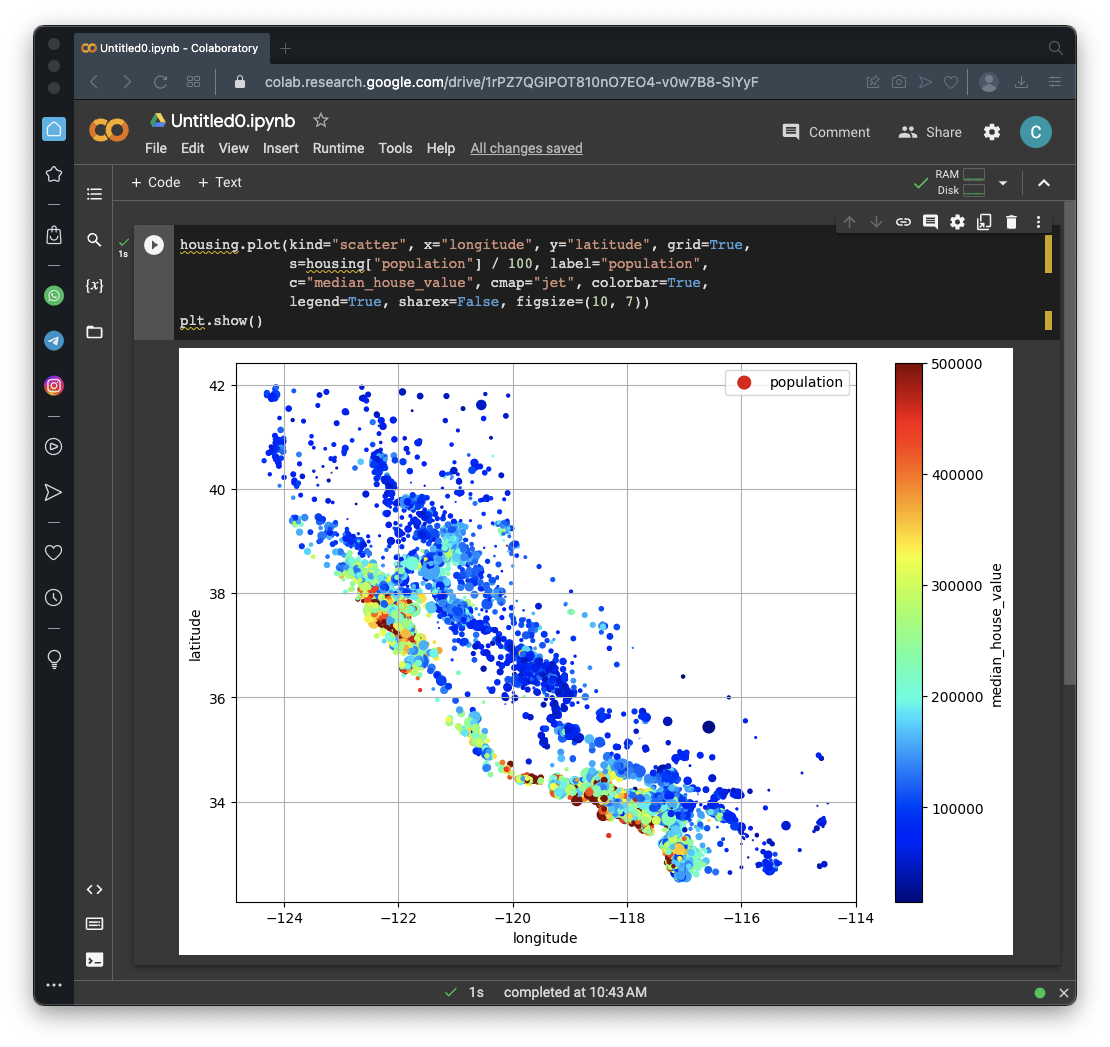## Correlations

Execute these commands to see how much each parameter correlates with median_house_value, by calculating the correlation coefficient "r". An r of 1 indicates the strongest possible correlation, and 0 means no correlation.
```corr_matrix = housing.corr() corr_matrix["median_house_value"].sort_values(ascending=False) ```
As shown below, median_income, total_rooms, and housing_median_age are all positively correlated with median_house_value (r > 0.1).## Scatter Plots

Execute these commands to see scatter plots of the important parameters:
```from pandas.plotting import scatter_matrix attributes = ["median_house_value", "median_income", "total_rooms", "housing_median_age"] scatter_matrix(housing[attributes], figsize=(12, 8)) plt.show() ```
There are four rows of plots, but we only care about the first row, as shown below.

The leftmost plot is a histogram of median_house_value, which we've seen before, and it's not helpful here.

The remaining three plots show how much the other parameters influence median_house_value, and make it obvious that median_income has a large effect, and the other two parameters have very little effect.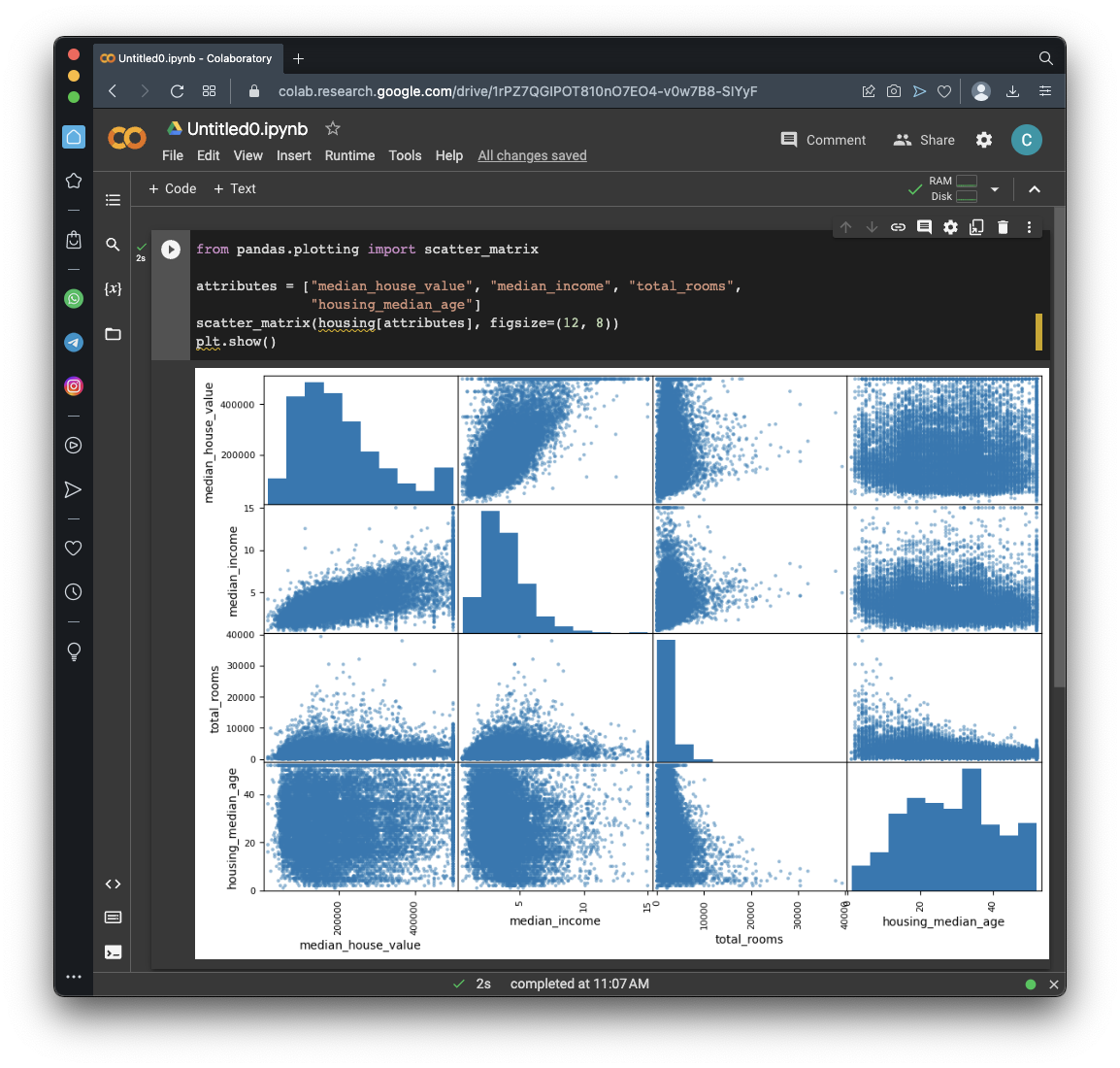Execute these commands to see the scatter plot of median_income and median_house_value in more detail:
```housing.plot(kind="scatter", x="median_income", y="median_house_value", alpha=0.1, grid=True) plt.show() ```
As shown below, there's a clear increasing trend. There are also artifacts--horizontal lines at 500,000, 450.000, 350,000, and possibly 280,000. Those look like strange exceptional cases in the original data.

It might be best to remove the districts on those lines from the data to prevent the model from learning to reproduce those artifacts which don't represent accurate inputs.## Attribute Combinations

Execute these commands to create three new columns combining attributes, and calculate the correlations:
```housing["rooms_per_house"] = housing["total_rooms"] / housing["households"] housing["bedrooms_ratio"] = housing["total_bedrooms"] / housing["total_rooms"] housing["people_per_house"] = housing["population"] / housing["households"] corr_matrix = housing.corr() corr_matrix["median_house_value"].sort_values(ascending=False) ```
As shown below,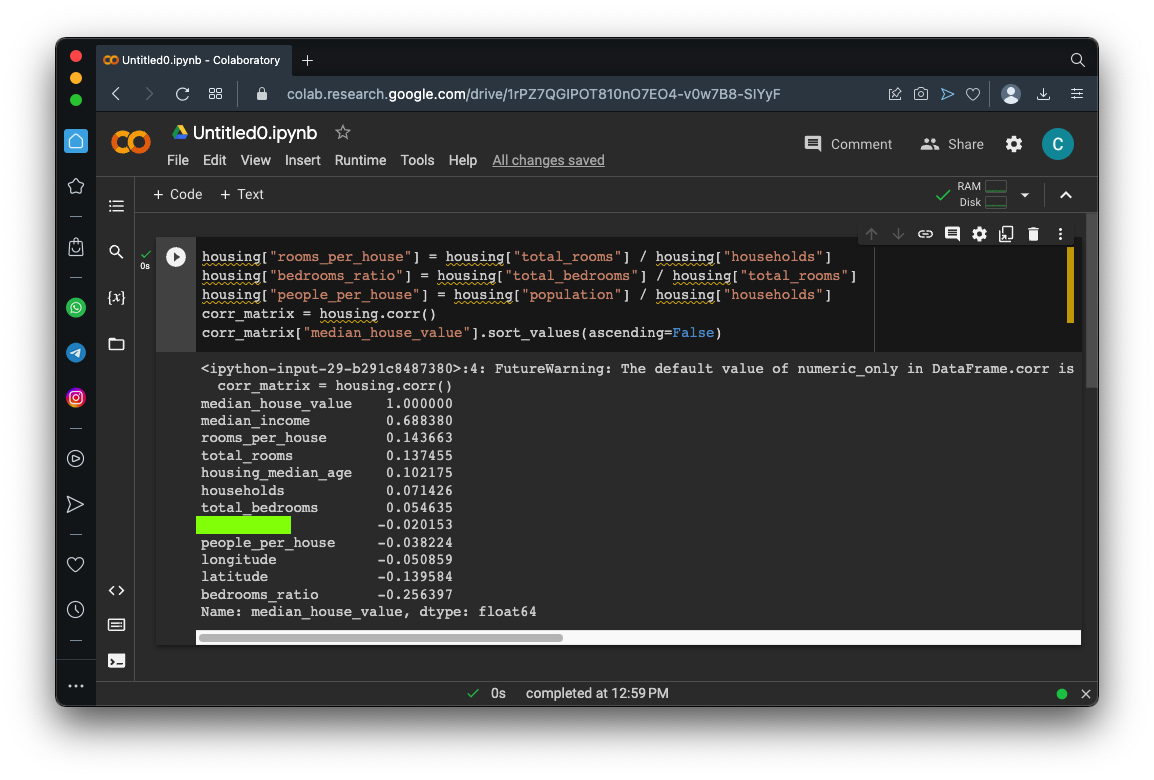## Flag ML 104.3: Attribute Combinations (5 pts)

Execute these commands to create three new columns combining attributes, and calculate the correlations:
```housing["rooms_per_house"] = housing["total_rooms"] / housing["households"] housing["bedrooms_ratio"] = housing["total_bedrooms"] / housing["total_rooms"] housing["people_per_house"] = housing["population"] / housing["households"] corr_matrix = housing.corr() corr_matrix["median_house_value"].sort_values(ascending=False) ```
As shown below, bedrooms_ratio has a strong negative correlation of -0.25, much larger than the correlations of total_bedrooms or total_rooms.

The flag is covered by a green rectangle in the image below.## Sources

Hands-On Machine Learning with Scikit-Learn, Keras, and TensorFlow 3rd Edition, Kindle Edition

Posted 4-13-23